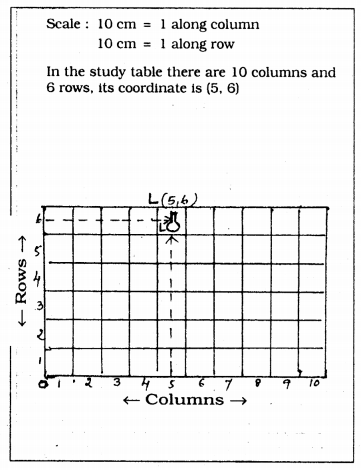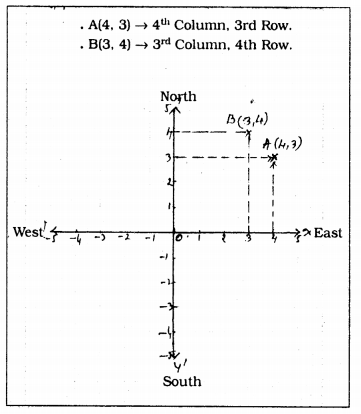# KSEEB Solutions for Class 9 Maths Chapter 9 Coordinate Geometry Ex 9.1

KSEEB Solutions for Class 9 Maths Chapter 9 Coordinate Geometry Ex 9.1 are part of KSEEB Solutions for Class 9 Maths. Here we have given Karnataka Board Class 9 Maths Chapter 9 Coordinate Geometry Exercise 9.1.

## Karnataka Board Class 9 Maths Chapter 9 Coordinate Geometry Ex 9.1

Question 1.
How will you describe the position of a table lamp on your study table to another person ?
Solution:
Length of study table = 100 cm.Scale : 10 cm = 1 row
Length of table 100 cm = 10 column.
Breadth 60 cm = 6 rows.

Question 2.
(Street Plan) : A city has two main roads which cross each other at the centre of the city. These two roads are along the North-South direction and East-West direction. All the other streets of the city-run parallel to these roads and are 200 m apart. There are 5 streets in each direction. Using 1 cm = 200 m, draw a model of the city in your notebook. Represent the roads/streets by single lines.
There are many cross-streets in your model. A particular cross-street is made by two streets, one running in the North-South direction and another in the East-West direction. Each cross street is referred to in the following manner:
If the 2nd street running in the North-South direction and 5th in the East-West direction meet at some crossing, then we will call this cross-street (2, 5). Using this convention, find:
(i) how many cross – streets can be referred to as (4, 3).
(ii) How many cross – streets can be referred to as (3, 4).
Solution:
Scale : 200 m = 1 cm.We hope the KSEEB Solutions for Class 9 Maths Chapter 9 Coordinate Geometry Ex 9.1 helps you. If you have any query regarding Karnataka Board Class 9 Maths Chapter 9 Coordinate Geometry Exercise 9.1, drop a comment below and we will get back to you at the earliest.